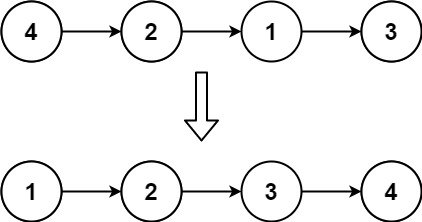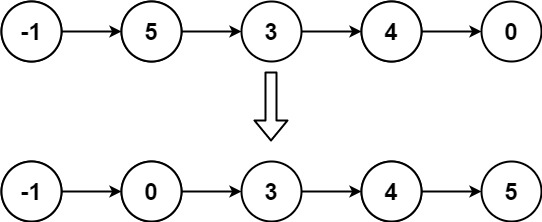147. Insertion Sort List

#### QUESTION:

Given the head of a singly linked list, sort the list using insertion sort, and return the sorted list’s head.

The steps of the insertion sort algorithm:

Insertion sort iterates, consuming one input element each repetition and growing a sorted output list. At each iteration, insertion sort removes one element from the input data, finds the location it belongs within the sorted list and inserts it there. It repeats until no input elements remain. The following is a graphical example of the insertion sort algorithm. The partially sorted list (black) initially contains only the first element in the list. One element (red) is removed from the input data and inserted in-place into the sorted list with each iteration.Example 1:``````Input: head = [4,2,1,3]
Output: [1,2,3,4]
``````

Example 2:``````Input: head = [-1,5,3,4,0]
Output: [-1,0,3,4,5]
``````

Constraints:

The number of nodes in the list is in the range [1, 5000]. -5000 <= Node.val <= 5000

#### SOLUTION:

``````class Solution {
func insertionSortList(_ head: ListNode?) -> ListNode? {
return nil
}

let dummy = ListNode(0)
var pre: ListNode? = dummy
var next: ListNode? = nil
// 新建一条list, 头是dummy, 然后按顺序往后排
while cur != nil {
next = cur?.next
// 按顺序查找当前的cur应该在的位置
while pre?.next != nil && pre!.next!.val < cur!.val {
pre = pre?.next
}
// 将cur插入到dummy为头的链中
cur?.next = pre?.next
pre?.next = cur
// 将pre重置为dummy头
pre = dummy
cur = next
}

return dummy.next
}
}
``````